# AP Physics C Electricity : Understanding Magnetic Fields and Charges

## Example Questions

### Example Question #1 : Understanding Magnetic Fields And Charges

A proton traveling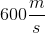enters a uniform magnetic field and experiences a magnetic force, causing it to travel in a circular path. Taking the magnetic field to be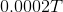, what is the radius of this circular path (shown in red)?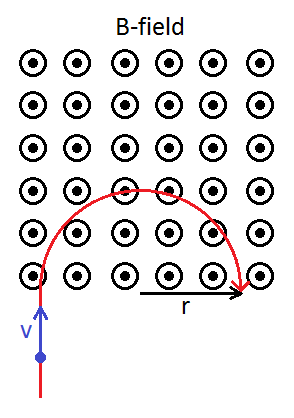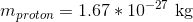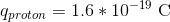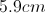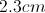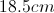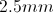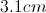Explanation:

To calculate the magnetic force of a single charge, we use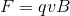, where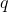is the charge of the proton,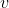is its velocity,is the uniform magnetic field.

Since this magnetic force causes the proton to travel in a circular path, we set this magnetic force equation equal to the centripetal force equation.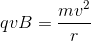is the mass of the proton andis the radius of the circular path. Solve for.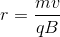Using the values given in the question, we can solve for the radius.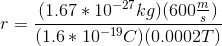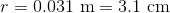### Example Question #181 : Ap Physics C

Which of the following best describes the net magnetic flux through a closed sphere, in the presence of a magnet?

Zero only if the magnet is completely enclosed within the surface

More than one of the other options is true

Negative only if the north pole of the magnet is within the surface

Zero regardless of the orientation of the magnet

Positive only if the north pole of the magnet is within the surface

Zero regardless of the orientation of the magnet

Explanation:

The net magnetic flux (or net field flowing in and out) through any closed surface must always be zero. This is because magnetic field lines have no starting or ending points, so any field line going into the surface must also come out. In other words, "there are no magnetic monopoles."

### Example Question #3 : Magnetism

A particle of charge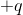and mass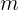moves with a speed ofperpendicular to a uniform magnetic field,. What is the period of the particle's orbit in the field?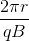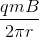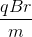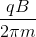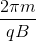Explanation:

Relevant equations: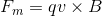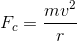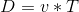Set the magnetic force equal to the centripetal force, since the magnetic force is directed towards the center of the particle's circular path and centripetal force is defined as the net force towards the center of a circular path.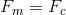Rearrange to isolate  the velocity: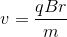Determine the distance,, traveled in one revolution, which is the circumference of a circle of radius: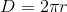Plug this distance and velocity into, to solve for the period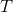: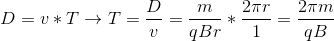### Example Question #4 : Magnetism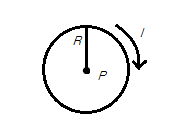Consider a current-carrying loop with current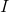, radius, and centerA particle with charge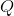flies through the center and into the page with velocity. What is the total electromagnetic force on the particle at the instant that it flies through the loop, in terms of the variables given?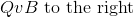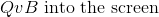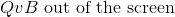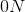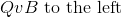Explanation:

The correct answer is zero. To calculate the force of a magnetic field on a moving charged particle, we use the cross product. We know that if the magnetic field is parallel to the velocity vector of the particle, then the force produced is zero.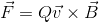Because our magnetic field in this case is going in the same direction as the velocity of the particle, we know that the magnetic force on the particle is zero.

### Example Question #5 : Magnetism

Consider two long, straight, current-carrying wires at distancefrom each other, each with a current of magnitudegoing in opposite directions.

If the two wires described are not held in place, what motion will result from the magnetic fields produced?

The wires will move toward each other

The wires will rotate counterclockwise

The wires will move away from each other

The wires will rotate clockwise

The wires will remain in place